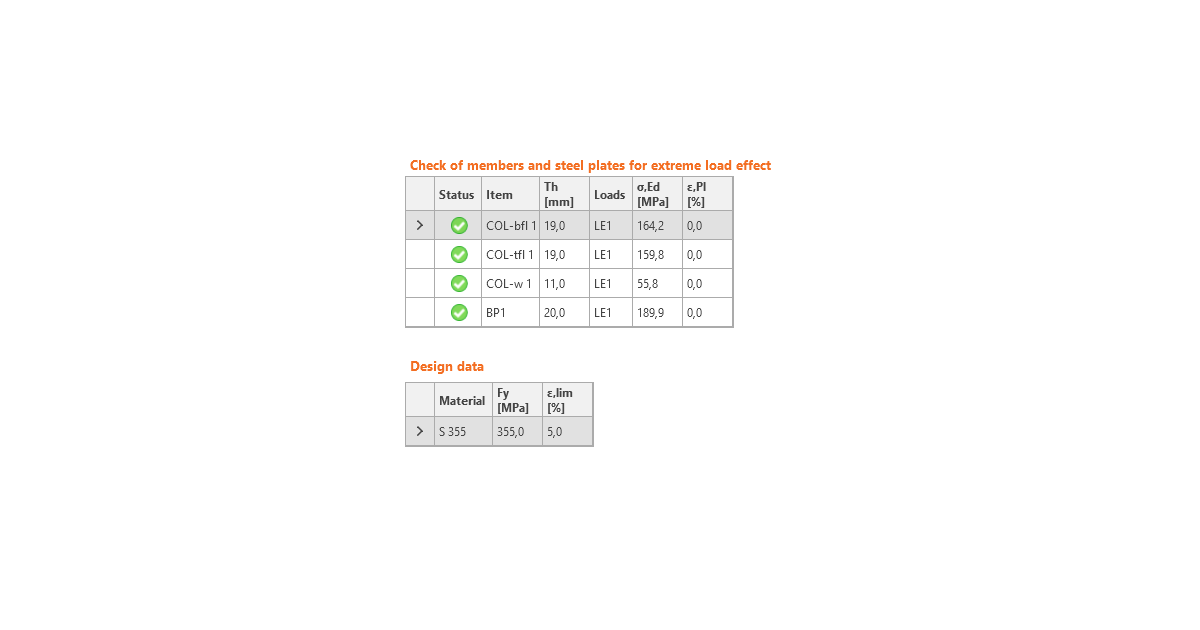### Choose language# Code-check of steel plates according to Russian standards

$$Strain check is performed at shell finite elements simulating plates. The yield strength is divided by the factor for material strength and multiplied by the service factor.The resulting equivalent stress (HMH, von Mises) and plastic strain are calculated on plates. When the yield strength (divided by partial safety factor for material strength, γm – SP 16, Table 3, and multiplied by service factor γc – SP 16, Table 1, which is editable in Code setup, SP 16, Cl. 11.1.1) on the bilinear material diagram is reached, the check of the equivalent plastic strain is performed. The limit value of 5 % is suggested in Eurocode (EN 1993-1-5 App. C, Par. C8, Note 1). This value can be modified in the Code setup, but verification studies were made for this recommended value. The material properties of the member are determined by the thickest plate.$\frac{1}{R_y \gamma_c} \sqrt{\sigma_x^2-\sigma_x \sigma_y + \sigma_y^2 + 3 \tau_{xy}^2} \le 1.0$The plate element is divided into five layers, and elastic/plastic behavior is investigated in each of them. The program shows the worst result from all of them.Stress may be a little bit higher than the design yield strength. The reason is the slight inclination of the plastic branch of the stress-strain diagram, which is used in the analysis to improve the stability of the calculation.$$